# V&V Article 1: Modeling Complex Frequency Selective Surfaces Using EM.Cube

Jump to: navigation, search
 V&V Project: Modeling Complex Frequency Selective Surfaces Using EM.Cube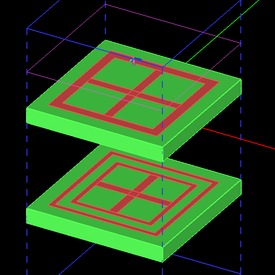Objective: In this article, different types of FSS structures are simulated using EM.Tempo and EM.Picasso, and the results are validated by the published data. Concepts/Features: EM.Tempo EM.Picasso Periodic Structures Plane Wave Source Reflection Coefficient Transmission Coefficient Minimum Version Required: All versions

## Introduction

In this verification & validation (V&V) article, we will model a number of frequency selective surface (FSS) structures using EM.Picasso and EM.Tempo, two of EM.Cube’s computational modules featuring planar method of moments (MOM) and finite difference time domain (FDTD) solvers, respectively. The simulation results of these solvers will be compared with the published simulated and measured data.

## Sandwiched Gridded Square Loop FSS

The first FSS structure to be simulated is a gridded square loop shown in Figure 1. The FSS elements are printed on a very thin Kapton substrate, which is sandwiched between two Teflon layers as shown in Figure 2. The following table shows the substrate properties as well as the geometrical dimensions of the gridded square loop structure:

Parameter Name Value Units
Kapton layer height (h1) 0.076 mm
Kapton permittivity (εr1) 3.5 -
Kapton loss tangent (tanδ1 @ 10GHz) 0.01 -
Teflon layer height (h2) 0.889 mm
Teflon permittivity (εr2) 2.2 -
Teflon loss tangent (tanδ2 @ 10GHz) 0.005 -
W1 0.4242 mm
W2 0.8510 mm
G 0.4242 mm
P 6.8 mm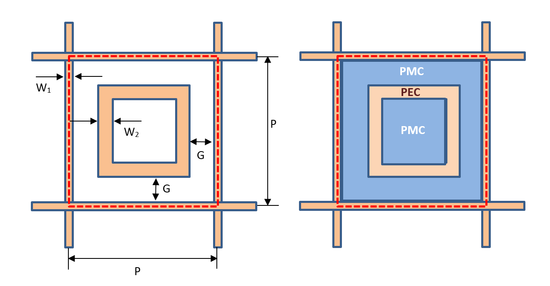Figure 1: Geometry of periodic unit cell for the gridded square loop FSS and it equivalent slot (PMC) model.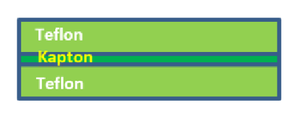Figure 2: The substrate layer hierarchy of the gridded square loop FSS.

In EM.Picasso, it is easier and computationally more efficient to model the above FSS unit cell using slot (PMC) traces. The diagram on the right side of Figure 1 shows the slot equivalent of the gridded square loop element. The dotted red line shows the boundary of the periodic unit cell structure. In this model, the top surface of the Kapton substrate is assumed to be covered with a PEC ground, on which two concentric slot traces (or apertures) are cut out: a rectangle at the center and a square loop enclosing it, as shown in Figure 1 in blue color. Figures 3 and 4 show the geometrical construction of the periodic unit cell of the gridded square loop FSS in EM.Tempo and EM.Picasso, respectively.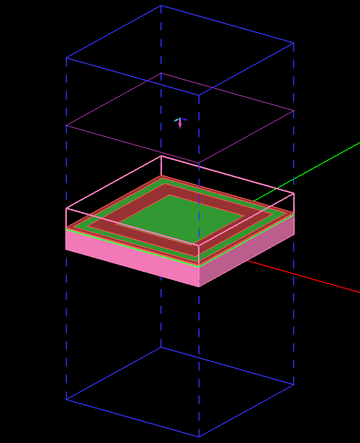Figure 3: Periodic unit cell structure setup for gridded square loop FSS in EM.Tempo. The top superstrate layer is shown in the freeze state.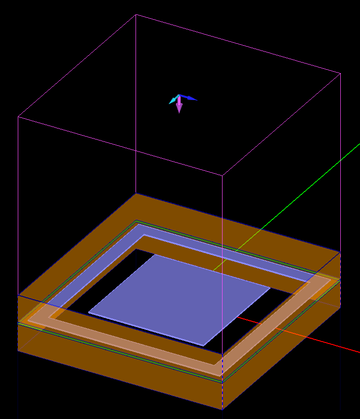Figure 4: Periodic unit cell structure setup for gridded square loop FSS in EM.Picasso. Note that the blue traces are slots in a horizontal PEC plane.

The gridded square loop FSS structure is excited using a plane wave source. First, the case of normal incidence is considered. For this case, Ref  does not provide measured data. However, it gives simulated data using the method of moments, which is the same technique used by EM.Picasso’s periodic planar simulator. At normal incidence (θ = 180°), the TE and TM polarizations must give the same results due to the X-Y symmetry of the unit cell structure. Figure 5 shows a comparison of the reflection coefficient data as computed by EM.Picasso and EM.Tempo. Figure 6 shows a comparison of the transmission coefficient data as computed by EM.Picasso, EM.Tempo and those given by Ref. .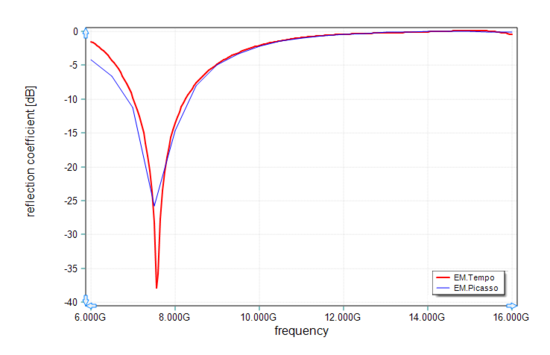Figure 5: A comparison of simulated reflection coefficient of the sandwich gridded square loop FSS with normal incidence computed by EM.Picasso (solid blue line) and EM.Tempo (solid red line).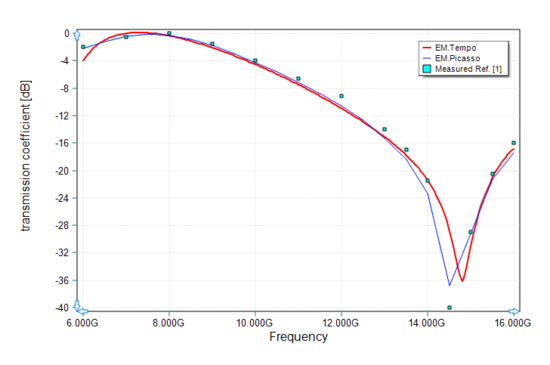Figure 6: A comparison of simulated transmission coefficient of the sandwich gridded square loop FSS with normal incidence computed by EM.Picasso (solid blue line), EM.Tempo (solid red line) and Ref.  (turquoise symbols).

Next, we consider the case of oblique incidence at 30° from the zenith for both TE and TM polarizations. In EM.Cube's standard spherical coordinate system, this oblique plane wave incidence corresponds to an incident angle of θ = 150°. Figures 7 and 8 compare the reflection and transmission coefficients, respectively, for the case of TE polarization, computed by EM.Picasso and EM.Tempo with the measured transmission data presented by Ref. . Figures 9 and 10 compare the reflection and transmission coefficients, respectively, for the case of TM polarization, computed by EM.Picasso and EM.Tempo with the measured transmission data presented by Ref. .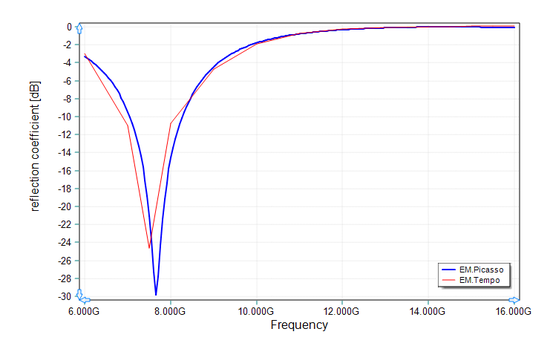Figure 7: A comparison of simulated reflection coefficient of the sandwich gridded square loop FSS for TE polarization and oblique incidence (30°) computed by EM.Picasso (solid blue line) and EM.Tempo (solid red line).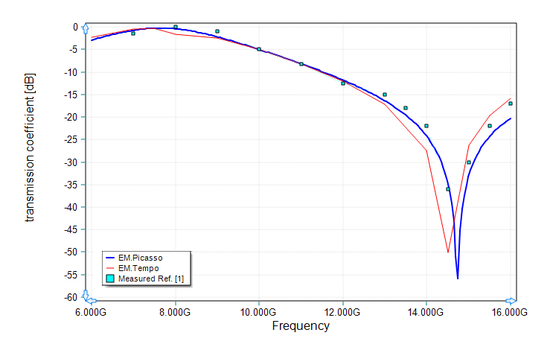Figure 8: A comparison of simulated and measured transmission coefficient of the sandwich gridded square loop FSS for TE polarization and oblique incidence (30°) computed by EM.Picasso (solid blue line), EM.Tempo (solid red line) and Ref.  (turquoise symbols).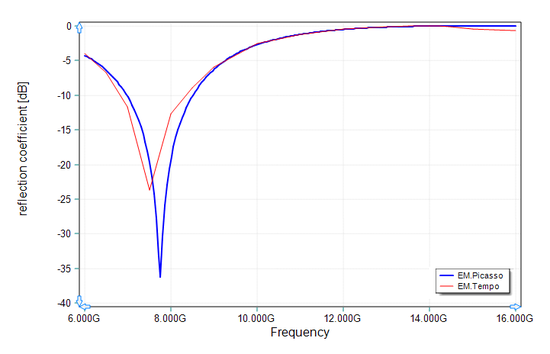Figure 9: A comparison of simulated reflection coefficient of the sandwich gridded square loop FSS for TM polarization and oblique incidence (30°) computed by EM.Picasso (solid blue line) and EM.Tempo (solid red line).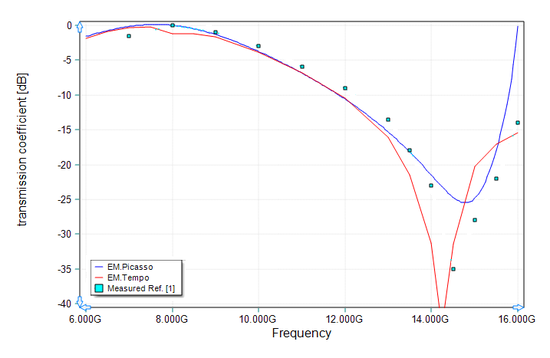Figure 10: A comparison of simulated and measured transmission coefficient of the sandwich gridded square loop FSS for TM polarization and oblique incidence (30°) computed by EM.Picasso (solid blue line), EM.Tempo (solid red line) and Ref.  (turquoise symbols).

## Double Square Loop FSS

The second FSS structure to be simulated consists of two concentric metallic square loops shown in Figure 11. The FSS elements are printed on a Duroid 6010.5 substrate. The following table shows the substrate properties as well as the geometrical dimensions of the double square loop structure:

Parameter Name Value Units
Duroid 6010.5 layer height (h1) 10 mils
Duroid permittivity (εr1) 11 -
Duroid loss tangent (tanδ1 @ 10GHz) 0.001 -
W1 5 mils
W2 21 mils
G1 10.5 mils
G2 21 mils
P 173 mils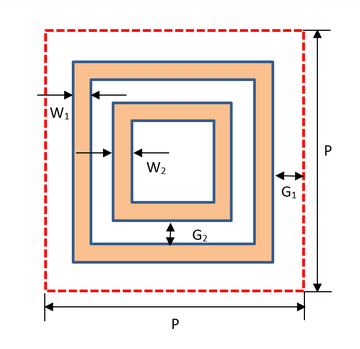Figure 11: Geometry of periodic unit cell for the double square loop FSS.

Similar to the previous case, the double square loop FSS structure is excited using a plane wave source. First, the case of normal incidence was considered. Figures 12 and 13 show the periodic unit cell structure setup in EM.Picasso and EM.Tempo, respectively.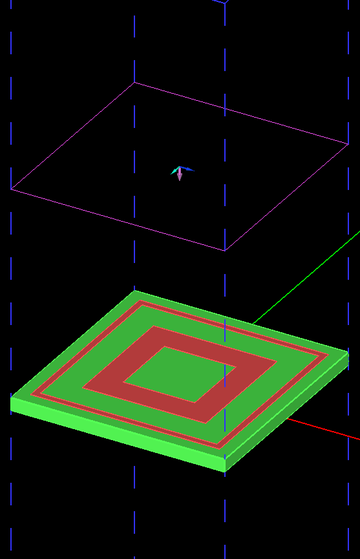Figure 12: Periodic unit cell structure setups for the double square loop FSS in EM.Tempo.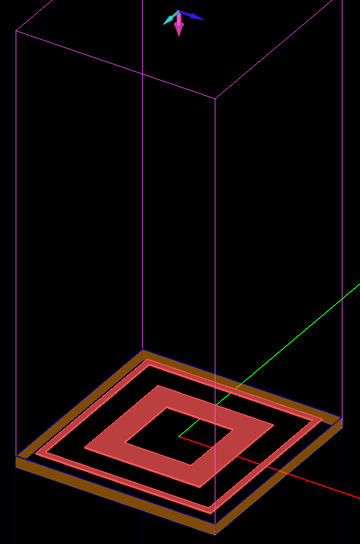Figure 13: Periodic unit cell structure setups for the double square loop FSS in EM.Picasso.

Figure 14 shows a comparison of the reflection coefficient data as computed by EM.Picasso and EM.Tempo. For this case, Ref  does provide measured transmission data at normal incidence. Figure 15 shows a comparison of the transmission coefficient data as computed by EM.Picasso, EM.Tempo and those given by Ref. .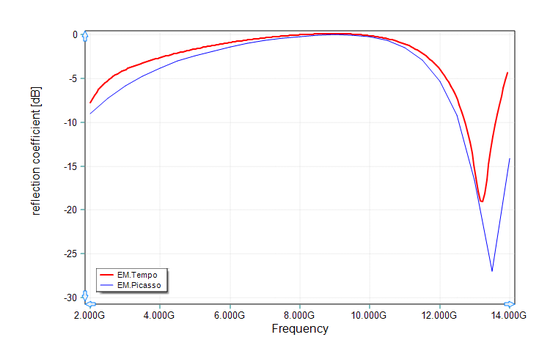Figure 14: A comparison of simulated reflection coefficient of the double square loop FSS with normal incidence computed by EM.Picasso (solid blue line) and EM.Tempo (solid red line).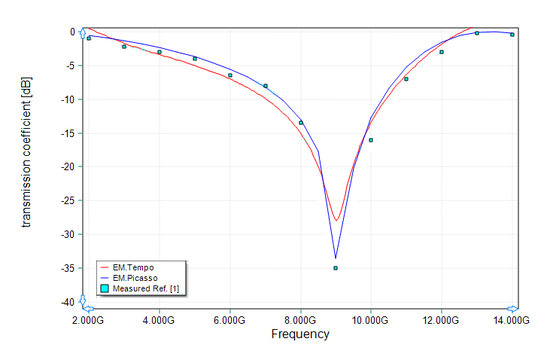Figure 15: A comparison of simulated and measured transmission coefficient of the double square loop FSS with normal incidence computed by EM.Picasso (solid blue line), EM.Tempo (solid red line) and Ref.  (turquoise symbols).

## Wideband Dual Screen FSS

The next FSS structure to be simulated is a wideband square loop shown in Figure 16. The FSS elements are printed on two thin FR4 substrates separated from each other with a spacing of 9.5mm. The following table shows the substrate properties as well as the geometrical dimensions of the wideband dual screen structure:

Parameter Name Value Units
FR4 layer height (h1) 1.6 mm
FR4 permittivity (εr1) 4.4 -
FR4 loss tangent (tanδ1 @ 10GHz) 0.001 -
D 9.5 mm
X 15 mm
Y 15 mm
a1 10 mm
a2 8 mm
b 12 mm
s 12 mm
g 0.9 mm
d 1 mm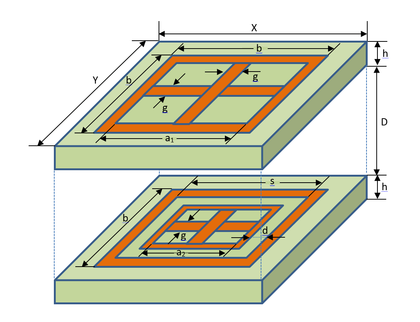Figure 16: Geometry of periodic unit cell for the wideband dual screen FSS.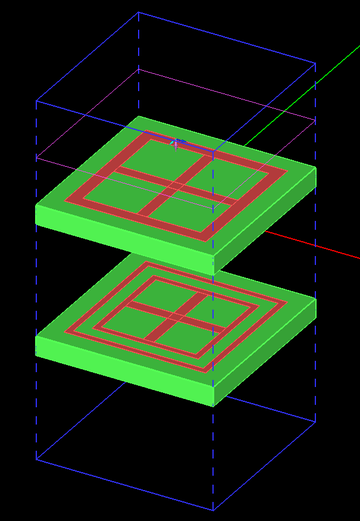Figure 17: Periodic unit cell structure of wideband dual screen FSS in EM.Tempo.

Figure 17 shows the periodic unit cell setup for the wideband dual screen FSS in EM.Tempo. The periodic structure is excited using a normally incident plane wave source, for which both TE and TM polarizations give the same results due to the symmetry of the unit cell geometry. Figure 18 shows a comparison of the simulated transmission coefficient data by EM.Tempo and the measured data given by Ref. .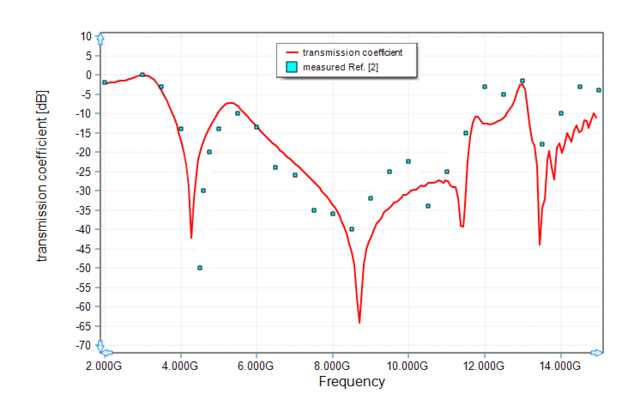Figure 18: A comparison of simulated and measured transmission coefficient of the wideband dual screen FSS with normal incidence computed by EM.Tempo (solid red line) and Ref.  (turquoise symbols).

## Double Ring FSS with a Triangular Lattice

The last FSS structure to be simulated consists of two concentric rings shown in Figure 19. The FSS elements are printed on a Duroid 6010.5 substrate. The following table shows the substrate properties as well as the geometrical dimensions of the double square loop structure:

Parameter Name Value Units
Duroid 6010.5 layer height (h1) 10 mils
Duroid permittivity (εr1) 11 -
Duroid loss tangent (tanδ1 @ 10GHz) 0.001 -
r1 100 mils
r2 35 mils
w1 4 mils
w2 34 mils
P 220 mils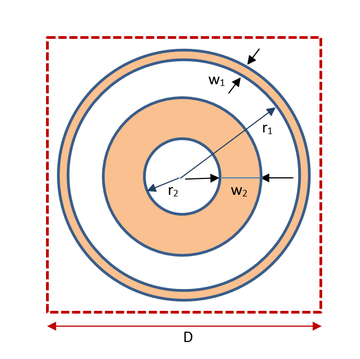Figure 19: Geometry of periodic unit cell for the double ring FSS.

The FSS structure in this case has an equilateral triangular (or hexagonal) lattice as shown below. This is known as a skewed grid. The location of each element is represented by the following equations:

$x_{mn} = m\Delta_x + n\Delta_x^{\prime}$
$y_{mn} = n\Delta_y + m\Delta_y^{\prime}$

where m and n are the integer indices, Δx and Δy are the primary periods and Δx‘ and Δy‘ are the secondary periods. For an equilateral triangular lattice, one has to set Δx = D and Δy = √3D/2, Δx‘ = D/2, and Δy‘ = 0, as shown in Figure 20. Figure 21 shows the periodic unit cell setup for the double ring FSS in EM.Picasso. Note that the outer ring extends beyond the boundaries of the periodic unit cell.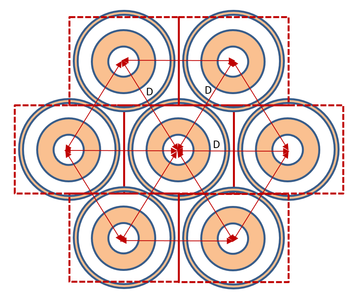Figure 20: The skewed grid of the double ring FSS.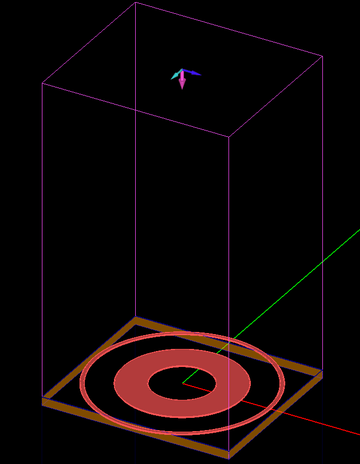Figure 21: Periodic unit cell structure setups for the double ring FSS in EM.Picasso.

This periodic structure is first excited using a normally incident plane wave source, for which both TE and TM polarizations give the same results due to the circular symmetry of the unit cell geometry. For normal incidence, Ref.  gives simulated results based on the method of moments. The same structure is then simulated using an obliquely incident plane wave source with an incident angle of θ = 150° (30° off the zenith) for both TE and TM polarization cases. Ref.  gives measured transmission data for both polarizations at this incident angle. Figure 22 shows a comparison of the simulated and measured transmission coefficient data for the double ring FSS. The simulated data by EM.Picasso correspond to both normal and oblique incidences and the data of Ref.  include simulated data for normal incidence and measured data for oblique incidence.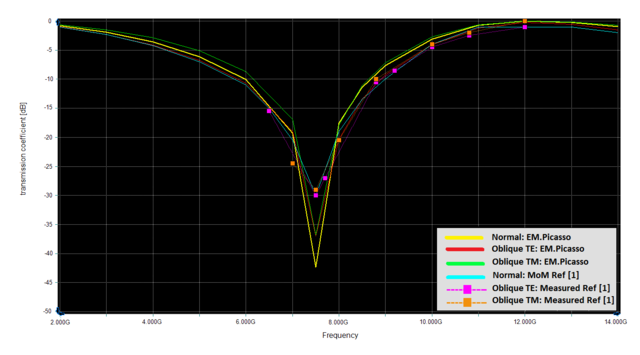Figure 22: A comparison of simulated and measured transmission coefficient of the double ring FSS. Solid yellow line: normal incidence computed by EM.Picasso, solid red line: oblique incidence with TE polarization computed by EM.Picasso, solid green line: oblique incidence with TM polarization computed by EM.Picasso, solid light blue line: normal incidence computed by Ref.  using MoM, pink symbols: measured data for oblique incidence with TE polarization given by Ref. , and orange symbols: measured data for oblique incidence with TM polarization given by Ref. .